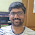## Gas Station

There are N gas stations along a circular route, where the amount of gas at station i is gas[i].
You have a car with an unlimited gas tank and it costs cost[i] of gas to travel from station i to its next station (i+1). You begin the journey with an empty tank at one of the gas stations.
Return the starting gas station's index if you can travel around the circuit once, otherwise return -1.
Note:
The solution is guaranteed to be unique.

### Analysis:

The key to this problem is "Don't make it too complex!"

Yes, after trying loop(which works but with high complexity), recursion, and DP, I failed at first. After reading the problem carefully, I forget the condition "unlimited gas tank"! Actually given this condition, this problem can be solved in one loop.

The idea is: consider the case that, if started at station i, and when goes to the station j, there is not enough gas to go the j+1 station. What happened now? For the brutal force method, we go back to the sataion i+1 and do the same thing. But, actually, if the accumutive gas cannot make it from j to j+1, then the stations from i to j are all not the start station. That is because, (1)the tank is unlimited, every time arrive to the station, the tank will fuel the max gas here, and comsume the cost to go to the next. (2)There can not be negative tank when arriving a station, at least the tank is empty. So, if i to j cannot go to j+1, then i+1 to j still cannot go to j+1... In this way, the next starting station we will try is not i+1, but the j+1. And after a single loop from i to j, we can find the result!

### Code:

class Solution {
public:
int canCompleteCircuit(vector<int> &gas, vector<int> &cost) {
int n = gas.size();
if (n==0){return -1;}
int i=0;
int j=0;
int tank=0;
while (true){
if (j>=n){return i;}
int net;
if (i+j>=n){
net = gas[i+j-n]-cost[i+j-n];
}else{
net = gas[i+j]-cost[i+j];
}
if (tank+net<0){
j++;
i=i+j;
if (i>=n){return -1;}
tank=0;
j=0;
}else{
tank+=net;
j++;
}
}
}
};


1.Consider the following test case
Fuel: { 3, 1, 2, 5, 4 }
Cost: {4, 1, 1, 2, 3 }

The output of your program is -1 for this input.

But here is the circuit which is possible if we start at index 1

From To Fuel(remaining fuel + current)
1 2 2
2 3 6
3 4 7
4 0 7
0 1 3

Did I understood the problem correct?

1.Your example is invalid since there are multiple valid start points (actually all indices except index 0 are valid), while the problem clearly specifies unique solution.

2.This comment has been removed by the author.

3.This comment has been removed by the author.

4.public static int numGas(int[] gas, int[] cost) {
int len = gas.length;
int sum = 0;
int start = 0;

for (int i = 0; i < len; i++) {
sum += gas[i];
sum -= cost[i];
if (sum < 0) {
start = i+1;
}
}

if (sum < 0) {
return -1;
}
return start;
}

5.//Simple solution using above concept

class Solution {
public:
int canCompleteCircuit(vector& gas, vector& cost) {
int len = gas.size();
int sum = 0;
int start = 0;
int i=0,j=0;
while(i=cost[i])
{
sum+=gas[i]-cost[i];
i++;
}
else
{
start=i+1;
sum=0;
i++;
}
}
if(j<0)
return -1;
return start;

}
};